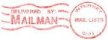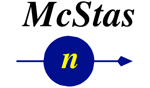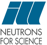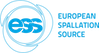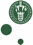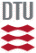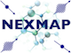McStas: SANS_Guinier Component

# The `SANS_Guinier` Component

Sample for Small Angle Neutron Scattering: Guinier model

## Identification

• Author: Henrich Frielinghaus
• Origin: FZ-Juelich/FRJ-2/IFF/KWS-2
• Date: Sept 2004
• Version:(Unknown)

## Description

```Sample that scatters with a Guinier shape. This is just an example where analytically
an integral exists. The neutron paths are proportional to the intensity
(low intensity > few paths).

Guinier function (Rg)
a = Rg*Rg/3
propability_unscaled = q * exp(-a*q*q)
integral_prop_unscal = 1/(2*a) * (1 - exp(-a*q*q))
propability_scaled   = 2*a * q*exp(-a*q*q) / (1 - exp(-a*q*q))
integral_prop_scaled = (1 - exp(-a*q*q)) / (1 - exp(-a*qmax*qmax))

In this simulation method many paths occur for high propability.
For simulation of low intensities see SANS_AnySamp.

Sample components leave the units of flux for the probability
of the individual paths. That is more consitent than the
Sans_spheres routine. Furthermore one can simulate the
transmitted beam. This allows to determine the needed size of
the beam stop. Only absorption has not been included yet to
these sample-components. But thats really nothing.

Example: SANS_Guinier(transm=0.5, Rg=100, qmax=0.03, xwidth=0.01, yheight=0.01, zdepth=0.001)

```
WARNING: This is a contributed Component.

## Input parameters

Parameters in boldface are required; the others are optional.
Name Unit Description Default
transm 1 (coherent) transmission of sample for the optical path "zdepth" 0.5
Rg Angs Radius of Gyration 100
qmax AA-1 Maximum scattering vector 0.03
xwidth m horiz. dimension of sample, as a width 0.01
yheight m vert.. dimension of sample, as a height 0.01
zdepth m thickness of sample 0.001

## Output parameters

Name Unit Description Default
q_v

• Source code for `SANS_Guinier.comp`.